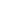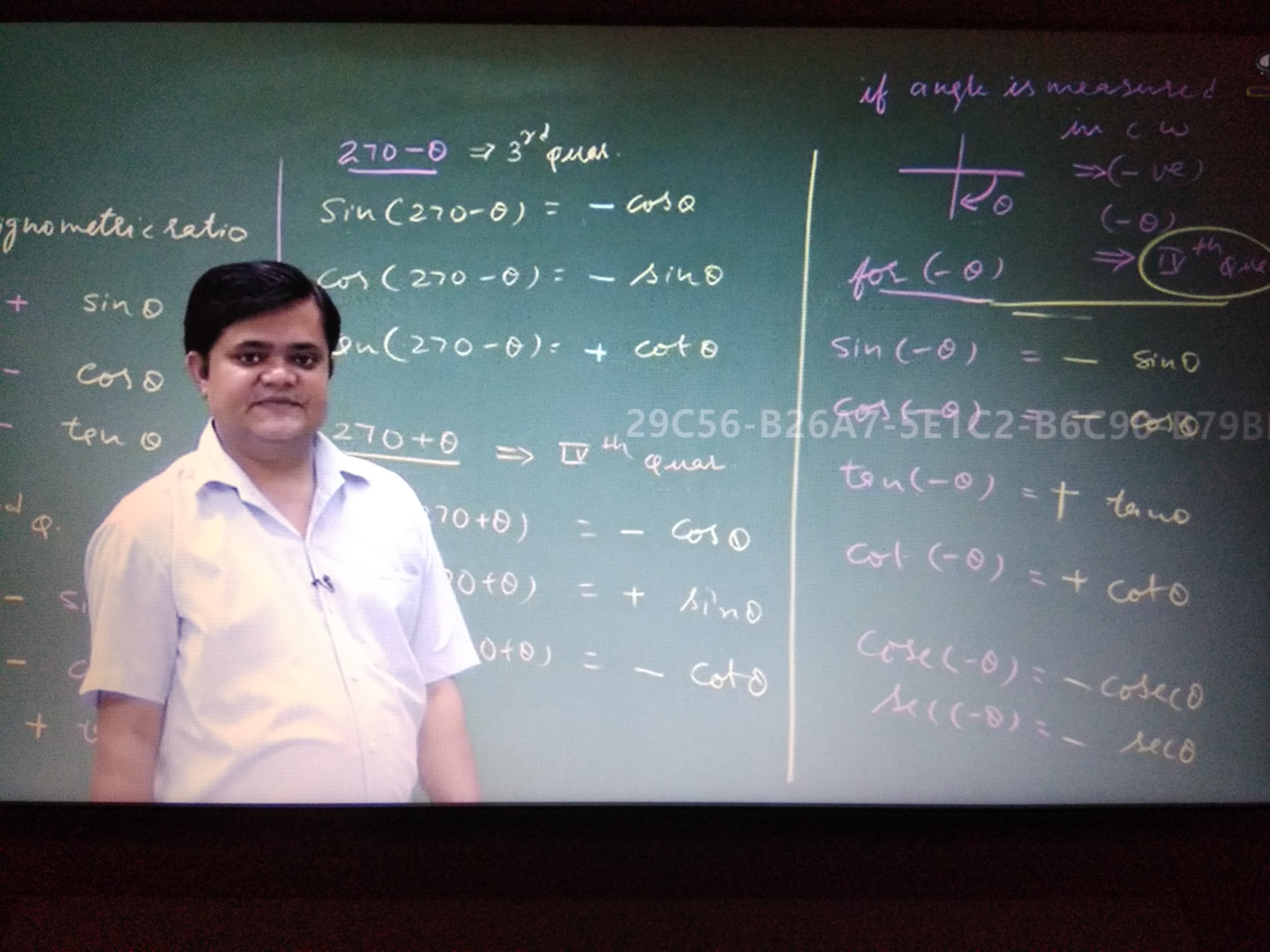Flat 50% off

Ends in

## If the angle is measured in c.w.direction then why are the measurement of the angle is in negative and start with the IV quadrant ? Why are the rules of measurement of angles of a.c.w direction is not applicable for this ? Sir please explain by giving some examples. Sir please look at the figure given below and check the formula for tan($-\theta$) and cot($-\theta$) is right or not??